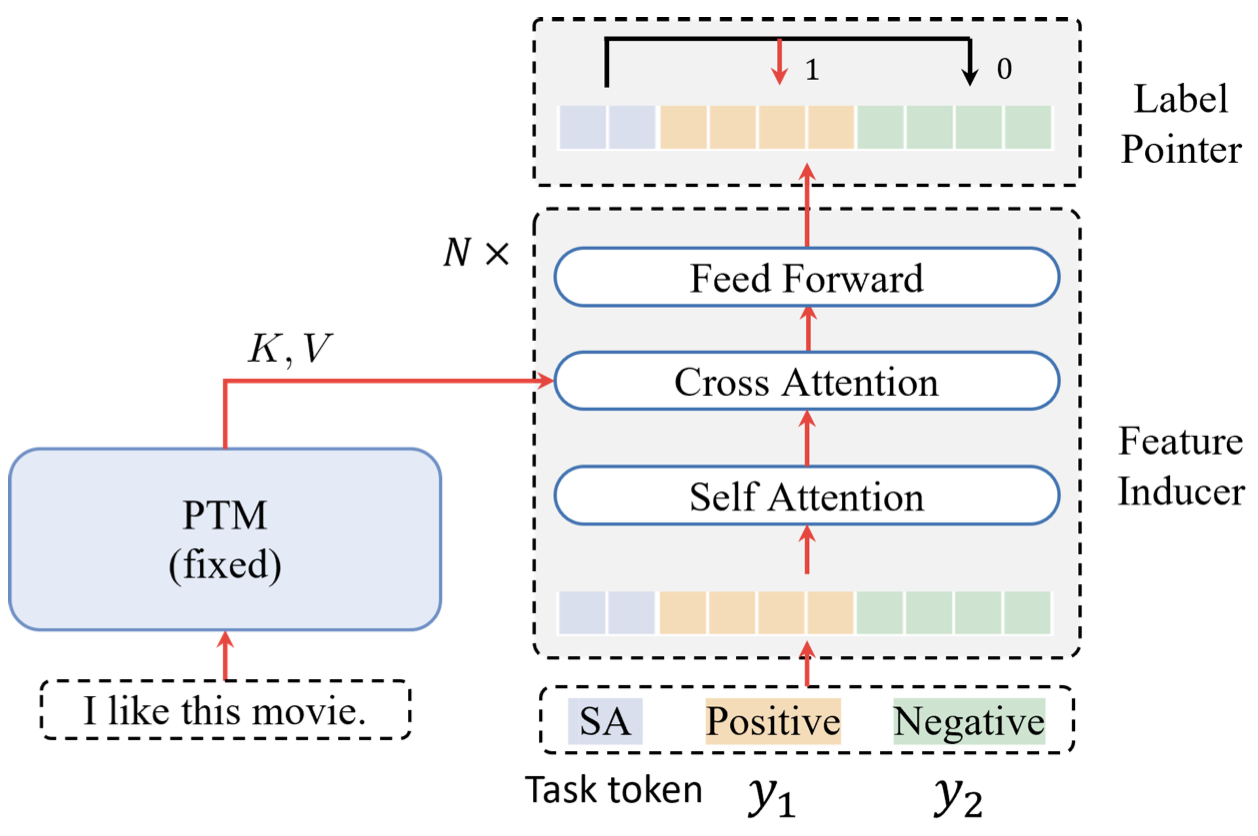# $\mathcal{Y}$-Tuning: 通过对标签表征进行微调的深度学习新范式

## December 13, 2021 • Read: 3921 • Deep Learning • 阅读设置

ACL2022有一篇名为《$\mathcal{Y}$-Tuning: An Efficient Tuning Paradigm for Large-Scale Pre-Trained Models via Label Representation Learning》的投稿，从标题上就吸引了我的注意，不同于Fine-Tuning、Adapter-Tuning、Prompt-Tuning，这篇文章的创新之处在于，不调整输入文本特征与大规模预训练模型的参数，仅通过学习标签的特征，以往很少见到论文去学习标签的特征。虽然最终效果可能仍无法与微调相比，但它在节省计算成本方面有很大的优势，并有可能通过后续研究进一步提高性能

### Preliminaries of Tuning PTMs

$$x = \text{I love this movie}$$

$$\phi, f = \mathop{\arg \min} \sum_{(x,y)\in \mathcal{D}} \ell (f(\phi (x)), y)\tag{1}$$

### $\mathcal{Y}$-TuningPTMs代表φ

\begin{aligned} \mathcal{L}_{\psi,f}(x,y) = &\sum_{y' \in \mathcal{Y}\atop y'\neq y}[f(\phi^{\star}(x), \psi(y^{\prime}))\\ &-f(\phi^{\star}(x), \psi(y)) + \alpha]_{+} \end{aligned}\tag{2}

$$\psi, f = \arg \min \sum_{(x,y)\in \mathcal{D}} \mathcal{L}_{\psi, f}(x,y)\tag{3}$$

$$\hat{y} = \mathop{\arg \max}_{y\in \mathcal{Y}}\ f(\phi^{\star}(x), \psi(y))\tag{4}$$

### An Implementation of $\mathcal{Y}$-Tuning

1. 用于提取文本特征的$\phi$，这个部分一般是Encoder类模型，例如BERT等
2. 用于提取标签特征的$\psi$，这个部分一般采用Transformer的Decoder结构，因为需要有Cross-Attention的部分对标签特征和文本特征进行交互
3. 用于预测类别的标签指针（Label Pointer），这个部分比较简单，用一个平均或者最大池化将高维向量转为低维向量即可

#### Label Embedding

$$\mathbf{Y} = [e_T; e_1^{(1)};···;e_1^{(c)}] \in \mathbb{R}^{N\times D}\tag{5}$$#### Self-Attention and Cross-Attention

$$\text{Att}(\mathbf{Q}, \mathbf{K}, \mathbf{V}) = \text{Softmax}\left(\frac{\mathbf{Q}\mathbf{K}^{\top}}{\sqrt{D_k}}\right)\mathbf{V}\tag{6}$$

$$\mathbf{Q}=\mathbf{Y}\mathbf{W}_q\in \mathbb{R}^{N\times D}\\ \mathbf{K}=\mathbf{X}\mathbf{W}_k\in \mathbb{R}^{M\times D}\\ \mathbf{V}=\mathbf{X}\mathbf{W}_v\in \mathbb{R}^{M\times D}$$

#### Label Pointer

$$\mathbf{h} = [\mathbf{h}_T, \mathbf{h}_1, ···,\mathbf{h}_C]\tag{7}$$

$$\mathcal{L}(x,y) = \sum_{c=1}^C [\mathbf{h}_T^{\top}\mathbf{h}_c - \mathbf{h}_{T}^{\top}\mathbf{h}_{c^{\star}} + \alpha]_{+}\tag{8}$$

### Result### 个人总结1.小老弟